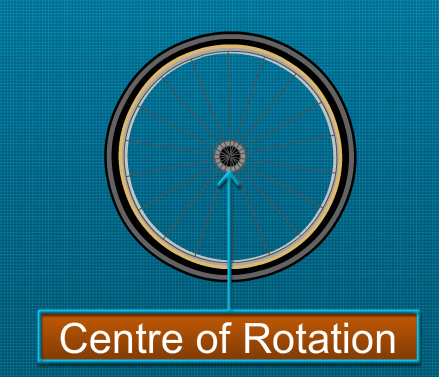Notes On Rotational Symmetry - CBSE Class 7 Maths
Any object or shape is said to have rotational symmetry if it looks exactly the same at least once during a complete rotation through 360°. Centre of rotation The fixed point about which an object rotates is called the centre of rotation. During the rotation, the shape and size of the object do not change. Rotation may be clockwise or anti-clockwise.A full turn refers to a rotation of 360°. A half turn refers to a rotation of 180°. A quarter turn refers to a rotation of 90°. Angle of rotation The angle at which a shape or an object looks exactly the same during rotation is called the angle of rotation. Order of rotational symmetry The order of rotational symmetry can be defined as the number of times that a shape appears exactly the same during a full 360o rotation. The centre of rotation of a square is the point of intersection of its diagonals and the angle of rotation is 90°. So, the order of rotational symmetry of a square is 4.The centre of rotation of a circle is the centre of the circle and it has rotational symmetry around the centre for every angle. There are many shapes that have only line symmetry and no rotational symmetry at all. Some objects and shapes have both, line symmetry as well as rotational symmetry. The Ashok Chakra in the Indian national flag has both, line symmetry and rotational symmetry.Symmetry can be seen in the English alphabet as well. The letter H has both line symmetry and rotational symmetry.          Letter    Line Symmetry           Rotational Symmetry           Z               No                        Yes           H               Yes                        Yes           O               Yes                        Yes           E               Yes                        No           N               No                        Yes           C               Yes                        No           A               Yes                        No           B               Yes                        No

#### Summary

Any object or shape is said to have rotational symmetry if it looks exactly the same at least once during a complete rotation through 360°. Centre of rotation The fixed point about which an object rotates is called the centre of rotation. During the rotation, the shape and size of the object do not change. Rotation may be clockwise or anti-clockwise.A full turn refers to a rotation of 360°. A half turn refers to a rotation of 180°. A quarter turn refers to a rotation of 90°. Angle of rotation The angle at which a shape or an object looks exactly the same during rotation is called the angle of rotation. Order of rotational symmetry The order of rotational symmetry can be defined as the number of times that a shape appears exactly the same during a full 360o rotation. The centre of rotation of a square is the point of intersection of its diagonals and the angle of rotation is 90°. So, the order of rotational symmetry of a square is 4.The centre of rotation of a circle is the centre of the circle and it has rotational symmetry around the centre for every angle. There are many shapes that have only line symmetry and no rotational symmetry at all. Some objects and shapes have both, line symmetry as well as rotational symmetry. The Ashok Chakra in the Indian national flag has both, line symmetry and rotational symmetry.Symmetry can be seen in the English alphabet as well. The letter H has both line symmetry and rotational symmetry.          Letter    Line Symmetry           Rotational Symmetry           Z               No                        Yes           H               Yes                        Yes           O               Yes                        Yes           E               Yes                        No           N               No                        Yes           C               Yes                        No           A               Yes                        No           B               Yes                        No

Next